Courses

# Facts That Matter- Polynomials Class 10 Notes | EduRev

## Class 10 : Facts That Matter- Polynomials Class 10 Notes | EduRev

The document Facts That Matter- Polynomials Class 10 Notes | EduRev is a part of the Class 10 Course Mathematics (Maths) Class 10.
All you need of Class 10 at this link: Class 10

Facts that Matter

• Polynomial
An algebraic expression of the form p(x) = a0 + a1 x + a2 x2 + a3 x3 + ...... + an xn, in which the variables involved have only non-negative integral exponents, is called a polynomial in x of degree n.

Note: In the polynomial a0 + a1 x + a2 x2 + .... + an xn
1. an ≠ 0
2. a0, a1 x1, a2 x2, a3 x3, ..... an - 1 xn - 1, anxn are terms.
3. a0, a1, a2, ..... an - 1, an are the co-efficients of x0, x1, x2, ....., xn-1, xn respectively.

• Degree of a Polynomial
The highest power of the variable in a polynomial is called its degree. For example,
p(x) = 5x + 3 is a polynomial in x of degree 1.
p(y) = 3y2 + 4y - 4 is a polynomial in y of degree 2.
• Linear Polynomial
A polynomial of degree 1 is called a linear polynomial. A linear polynomial is generally written as ax + b (a ≠ 0), where a, b are real coefficients.
A polynomial of degree 2 is called a quadratic polynomial. A quadratic polynomial is generally written as ax2 + bx + c (a ≠ 0), where a, b and c are real coefficients.
• Cubic Polynomial
A polynomial of degree 3 is called a cubic polynomial. A cubic polynomial is generally written as ax3 + bx2 + cx + d (a ≠ 0), where a, b, c and d are real coefficients.
Examples:
Degree of different polynomials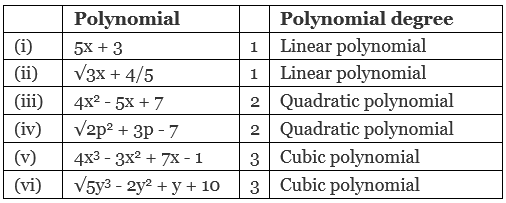• Value of a Polynomial at a Given Point
If p (x) is a polynomial in x and ‘a’ is a real number. Then the value obtained by putting
x = a in p (x) is called the value of p (x) at x = a.
Example:
Let p(x) = 5x2 - 4x + 2 then its value at x = 2 is given by
p (2) = 5 (2)2 - 4 (2) + 2
= 5 (4) - 8 + 2
= 20 - 8 + 2
= 14
Thus, the value of p(x) at x = 2 is 14.
• Zeroes of a Polynomial
A real number ‘a’ is said to be a zero of the polynomial p (x), if p (a) = 0.
Example:
Let p (x) = x2 - x - 2
Then p (2) = (2)2 - (2) - 2 = 4 - 4 = 0
and p (-1) = (-1)2 - (-1) - 2 = 2 - 2 = 0
∴ (-1) and (2) are the zeroes of the polynomial x- x - 2.

Note:
I. A linear polynomial has at the most one zero.
II. A quadratic polynomial has at the most two zeroes.

III. In general a polynomial of degree n has at the most n zeroes.

• Geometrical Meaning of the Zeroes of a Polynomial
First, we consider a linear polynomial p (x) = ax + b. Let ‘k’ be a zero, then
p (k) = ak + b = 0
⇒ ak + b = 0
⇒ ak = - b
or k =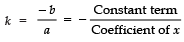The graph of a linear polynomial is always a straight line. It may or may not pass through
x-axis. In case the graph line is passing through a point on the x-axis, then the y-coordinate of that point must be zero.
In general, for a linear polynomial ax + b = 0, (a ≠ 0), the graph is a straight line which can intersect the x-axis at exactly one point, namely,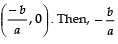is the zero of the polynomial ax + b.In the given figure, CD is meeting x-axis at x = -1.
∴ Zero of ax + b is -1.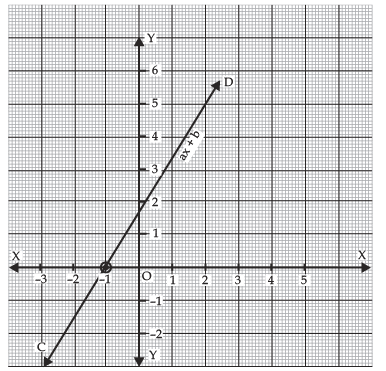Note:
A zero of a linear polynomial is the x-coordinate of the point, where the graph intersects the x-axis.

• Graph of a Quadratic Polynomial
The graph of ax2 + bx + c, (a ≠0) is a curve of ∪ shape, called a parabola. If a > 0 in
ax2 + bx + c, the shape of the parabola is ∪ (opening upwards). If a < 0 in ax2 + bx + c, the shape of parabola is ∩ (opening downwards).
In the given figure, the graph of a quadratic polynomial x2 - 3x - 4 is shown. It intersects x-axis at (-1, 0) and (4, 0). Therefore, its zeroes are -1 and 4. Here, a > 0, so the graph opens upwards.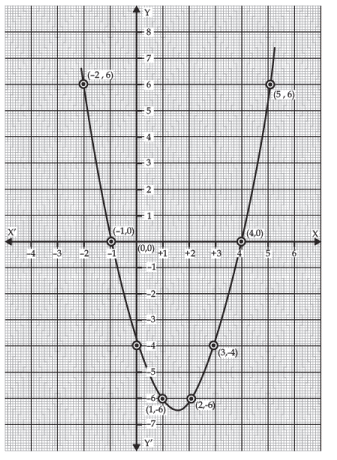Whereas the following figure is a graph of the polynomial - x2 + x + 6. Since it intersects the x-axis at (3, 0) and (-2, 0). Therefore, the zeroes of - x2 + x + 6 are -2 and 3.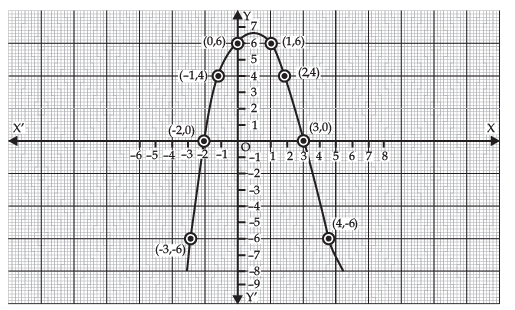Here a < 0, so the parabola opens downwards.
Offer running on EduRev: Apply code STAYHOME200 to get INR 200 off on our premium plan EduRev Infinity!

## Mathematics (Maths) Class 10

178 videos|268 docs|103 tests

,

,

,

,

,

,

,

,

,

,

,

,

,

,

,

,

,

,

,

,

,

;